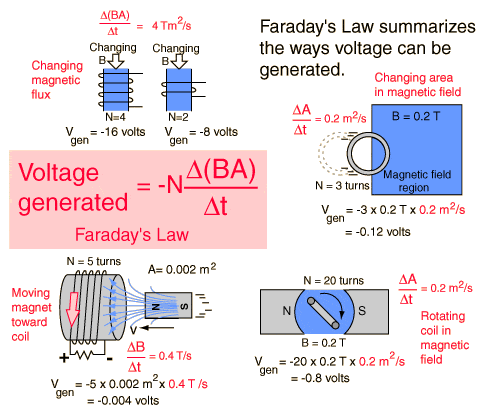# Equation For Electrical Production

AnAverageJoey
SO i am trying to figure out how much electricity can be produced through elector magnetism.

I figure the information needed would be

1.Density of the magnetic field.
2. The # of coils in the solenoid
3. The rate at which the solenoid passes over the magnetic field.
4. The distance the solenoid is from the magnetic field
5. The angle at which the solenoid comes in contact with the magnetic field

Am i missing any? and is there an equation that knowing these values i could calculate the amount of electricity produced. Or visa versa Knowing how much electricity i need to produce but be able to figure out the density of the magnetic field i would need to produce that amount of electricity. all the help i could get would be super duper,

Thanks, AnAverageJoey

Gold Member
Welcome to Physics Forums. What you need is to understand Faraday's Law.AnAverageJoey
So to generate 240 volts from a solenoid with 4 coils that passes over an unknown magnetic field at 29m/s it would take a magnetic field with a density of 2.0689 T?

Represented by 240v= (4)(x)(29) where x is the density of the magnetic field?

Ik it sounds like a homework question but its not just a personal project question lol